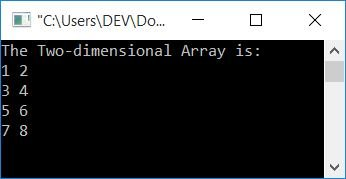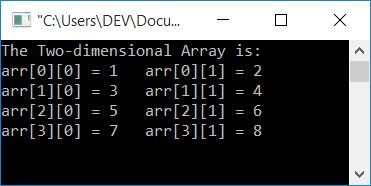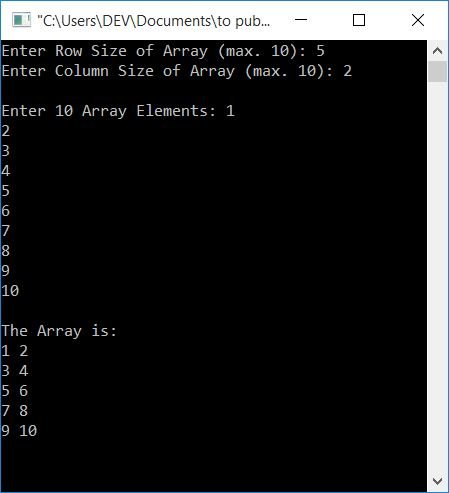# C Program for Two-Dimensional Array

In this article, you will learn about and get code for some programs that use two-dimensional (2D) arrays. For example,

`int arr = {{1, 2}, {3, 4}, {5, 6}, {7, 8}};`

is a two-dimensional array. The array's name is arr, and it contains four pairs of 2-2 elements.That is, the first size tells the total number of pairs, and the second size tells how many elements go into each pair. All the numbers from 1 to 8 get stored in the array arr[][] in a way that

• 1 is saved at arr.
• 2 is saved at arr.
• 3 is saved at arr.
• 4 is saved at arr.
• and so on.

You can also think of it as the elements of a two-dimensional array being arranged in rows and columns.That is, the first index shows rows, whereas the second one shows columns. So from the above statement, where the array arr[][] is declared and defined, it shows that there are 4 rows and 2 columns. Now let's move on to the program given below.

## Two-Dimensional Array Program in C

This is the simplest C program that uses a two-dimensional array. Here, the elements of the two-dimensional array get initialized automatically while running the program. That is, it does not ask the user for assistance with anything, such as defining the size or entering some elements for a 2D array. Let's have a look at the program.

```#include<stdio.h>
#include<conio.h>
int main()
{
int arr = {{1, 2}, {3, 4}, {5, 6}, {7, 8}};
int i, j;
printf("The Two-dimensional Array is:\n");
for(i=0;  i<4; i++)
{
for(j=0; j<2; j++)
printf("%d ", arr[i][j]);
printf("\n");
}
getch();
return 0;
}```

This program was built and runs in the Code::Blocks IDE. Here is the output produced by the above program:because the array size is defined as arr. That means we have four pairs. And each pair contains two elements. So here we have first created a for loop that runs four times, and then inside it, we have created another for loop that runs twice every time program flow enters this loop after coming from the outer loop. In this way, the statement,

`printf("%d ", arr[i][j]);`

executes a total of 8 times, with its index value starting from arr to arr. Let's verify it using the program given below.

You can also replace the above program with the one below to print the array's elements as well as their indexing. In other words, which element is stored at which index. Here is the program:

```#include<stdio.h>
#include<conio.h>
int main()
{
int arr = {{1, 2}, {3, 4}, {5, 6}, {7, 8}};
int i, j;
printf("The Two-dimensional Array is:\n");
for(i=0;  i<4; i++)
{
for(j=0; j<2; j++)
printf("arr[%d][%d] = %d\t", i, j, arr[i][j]);
printf("\n");
}
getch();
return 0;
}```

And here is its output:### Allow the user to define the array size

Consider another program in which the user can specify the size of a two-dimensional array and then enter elements of that size. This is the final program on 2D dimensional arrays that will give you a thorough understanding of them:

```#include<stdio.h>
#include<conio.h>
int main()
{
int arr, row, col, i, j;
printf("Enter Row Size of Array (max. 10): ");
scanf("%d", &row);
printf("Enter Column Size of Array (max. 10): ");
scanf("%d", &col);
printf("\nEnter %d Array Elements: ", row*col);
for(i=0; i<row; i++)
{
for(j=0; j<col; j++)
scanf("%d", &arr[i][j]);
}
printf("\nThe Array is:\n");
for(i=0; i<row; i++)
{
for(j=0; j<col; j++)
printf("%d ", arr[i][j]);
printf("\n");
}
getch();
return 0;
}```

The snapshot given below shows the sample run of the program that is given just above this paragraph:#### The same program in different languages

C Quiz

« Previous Program Next Program »